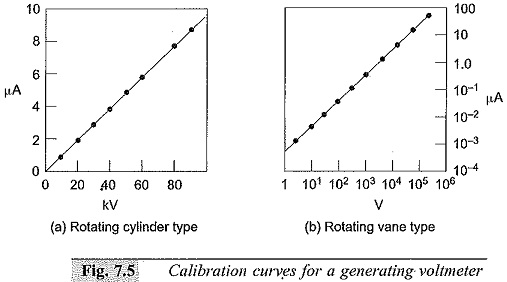## Generating Voltmeter Principle and Construction:

High voltage measuring devices employ generating principle when source loading is prohibited (as with Van de Graaff generators, etc.) or when direct connection to the high voltage source is to be avoided. A Generating Voltmeter Principle and Construction is a variable capacitor electrostatic voltage generator which generates current proportional to the applied external voltage. The device is driven by an external synchronous or constant speed motor and does not absorb power or energy from the voltage measuring source.

### Principle of Operation:

The charge stored in a capacitor of capacitance C is given by q = CV. If the capacitance of the capacitor varies with time when connected to the source of voltage V, the current through the capacitor,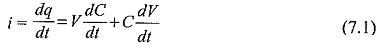For d.c. voltages dV/dt = 0. Hence,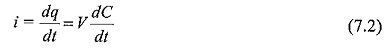If the capacitance C varies between the limits C0 and (C0 + Cm) sinusoidally as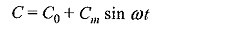the current i is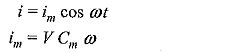(im, is the peak value of the current). The rms value of the current is given by: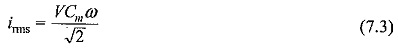a constant angular frequency of ω the current is proportional to the applied voltage V. More often, the generated current is rectified and measured by a moving coil meter. Generating Voltmeter Principle and Construction can be used for a.c voltage measurements also provided the angular frequency ω is the same or equal to half that of the supply frequency.

A Generating Voltmeter Principle and Construction with a rotating cylinder consists of two exciting field electrodes and a rotating two pole armature driven by a synchronous motor at a constant speed n. The a.c. current flowing between the two halves of the armature is rectified by a commutator whose arithmetic mean may be calculated from: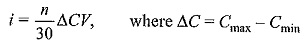For a symmetric voltage Cmin = 0. When the voltage is not symmetrical, one of the electrodes is grounded and Cmin has a finite value. The factor of proportionality n/30. ΔC is determined by calibration.

This device can be used for measuring a.c. voltages provided the speed of the drive-motor is half the frequency of the voltage to be measured. Thus a four-pole synchronous motor with 1500 rpm is suitable for 50 Hz. For peak value measurements, the phase angle of the motor must also be so adjusted that Cmax and the crest value occur at the same instant.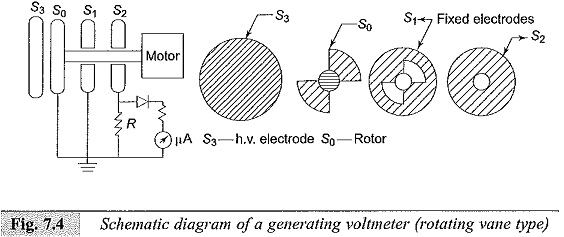Generating Voltmeter Principle and Construction employ rotating sectors or vanes for variation of capacitance. Figure 7.4 gives a schematic diagram of a generating voltmeter. The high voltage source is connected to a disc electrode S3 which is kept at a fixed distance on the axis of the other low voltage electrodes S0,S1 and S2 . The The rotor S0 is driven at a constant speed by a synchronous motor at a suitable speed (1500, 1800, 3000, or 3600 rpm). The rotor vanes of S0 cause periodic change in capacitance between the insulated disc S2 and the h.v. electrode S3. The shape and number of the vanes of S0 and S1 are so designed that they produce sinusoidal variation in the capacitance. The generated a.c. current through the resistance R is rectified and read by a moving coil instrument. An amplifier is needed if the shunt capacitance is large or longer leads are used for connection to rectifier and meter. The instrument is calibrated using a potential divider or sphere gap. The meter scale is linear and its range can be extended by extrapolation. Typical calibration curves of a Generating Voltmeter Principle and Construction are given in Figs. 7.5a and b.